# Constructions-NCERT Solutions

Class X Math
NCERT Solution for Constructions
NCERT TEXTBOOK QUESTIONS SOLVED
EXERCISE 11.1
In each of the following, give the justification of the construction also:
Q.1.   Draw a line segment of Length 7.6 cm and divide it in the ratio 5 : 8. Measure the two parts.
Sol. Steps of construction:
I. Draw a line segment AB = 7.6 cm.
II. Draw a ray AX making an acute angle with AB.
III. Mark 13 (8 + 5) equal points on AX, and mark them as X1, X2, X3, ........, X13.
IV. Join 'point X13' and B.
V. From 'point X5', draw X5C || X13B, which meets AB at C.
Thus, C divides AB in the ratio 5 : 8
On measuring the two parts, we get:
C = 4.7 cm and BC = 2.9 cm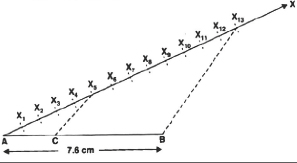Justification:
In Δ ABX13 and Δ ACX5, we have
CX5 || BX13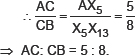Q.2.   Construct a triangle of sides 4 cm, 5 cm and 6 cm and then a triangle similar to it whose sides 2 are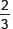of the corresponding sides of the first triangle.
Sol. Steps of construction:
I. Draw a Δ ABC such that BC = 6 cm, AC = 5 cm and AB = 4 cm.
II. Draw a ray BX making an acute angle ∠CBX.
III. Mark three points X1, X2, X3 on BX such that BX1 = X1X2 = X2X3.
IV. Join X3 C.
V. Draw a line through X2 such that it isss parallel to X3 C and meets BC at C.
VI. Draw a Iine through C parallel to CA to intersect BA at A'.
Thus, A'BC is the required triangle.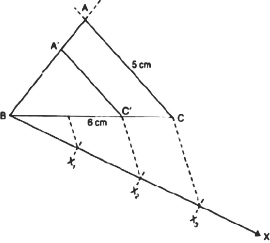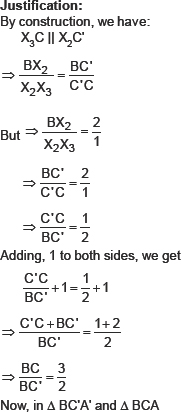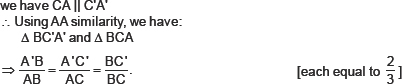Q.3.   Construct a triangle with sides 5 cm, 6 an and 7 cm and then another triangle whose sides are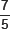of the corresponding sides of the first triangle.
Sol. Steps of construction:
I. Construct a Δ ABC such that AB = 5 cm, BC = 7 cm and AC = 6 cm.
II. Draw a ray BX such that ∠CBX is an acute angle.
III. Mark 7 points of X1, X2, X3, X4, X5, = X5X6 = X6X7
IV. Join X5 to C.
V. Draw a line through X7 intersecting BC (produced) at C' such that X5C || X7C'
VI. Draw a line through C' parallel to CA to intersect BA (produced) at A'. Thus, ΔA'BC' is the required triangle.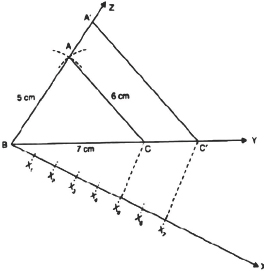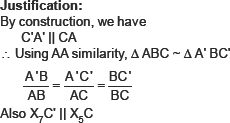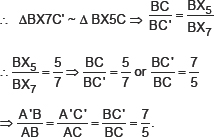Q.4.   Construct an isosceles triangle whose base is 8 an and altitude 4 cm and then another triangle whose sides are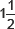times the corresponding sides of the isosceles triangle.
Sol. Steps of construction:
I. Draw BC = 8 cm
II. Draw the perpendicular bisector of BC which intersects BC at D.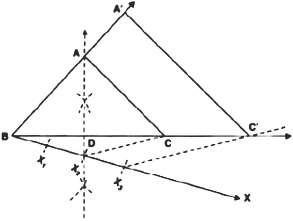III. Mark a point A on the above perpendicular such that DA = 4cm.
IV. Join AB and AC.
Thus, Δ ABC is the required isosceles triangle.
V. Now, draw a ray BX such that ∠CBX is an acute angle.
Vl. On BX, mark three points X1, X2 and X3 such that:
BX1 = X1X2 = X2X3
VII. Join X2 to C.
VIII. Draw a line through X3 parallel to X2 C and intersecting BC (extended) to C
IX. Draw a line through C' parallel to CA intersecting BA (extended) at A', thus, Δ A'BC' is the required triangle.
Justification:
We have C'A' || CA                                                        [By construction]
∴ Using AA similarity, Δ ABC ~ Δ A' BC'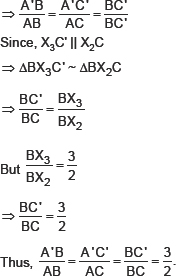Q.5.   Draw a triangle ABC with side BC = 6 cm, AB = 5 cm and ∠ABC = 60°. Don construct a triangle whose sides are 3 of the corresponding sides of the triangle ABC.
Sol. Steps of construction:
I. Construct a Δ ABC such that BC = 6 cm, AB = 5 cm and ∠ABC = 60°.
II. Draw a ray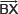such that ∠CBX is an acute angle.
III. Mark four points X1, X2, X3 and X4 on BX such that
BX1 = X1X2 = X2X3 = X3X4
IV. Join X4C and draw X3C' II X4C such that C' is on BC.
V. Also draw another line through C' and parallel to CA to intersect BA at A'.
Thus, Δ A'BC' is the required triangle.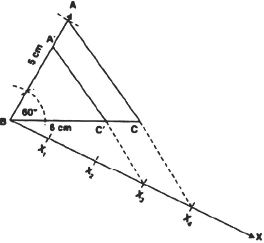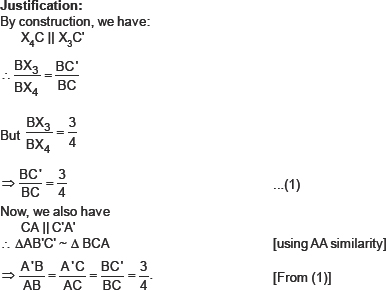Q.6.   Draw a triangle ABC with side BC = 7 cm, ∠B = 45°, ∠A = 105°. Then, construct a triangle whose sides are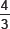times the corresponding sides of Δ ABC.
Sol. Steps of construction:
I. Construct a a ABC such that BC = 7 cm, LB = 45° and LA = 105°.
II. Draw a ray BX making an acute angle ∠CBX with BC.
III. On BX, mark four points X1, X2, X3 and X4 such that
BX1 = X1X2 = X2X3 = X3X4.
IV. Join X3 to C.
V. Draw X4C || X3C such that C' lies on BC (extended).
VI. Draw a line through C' parallel to CA intersecting the extended line segment BA at A'.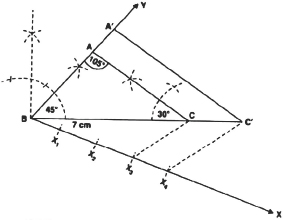Thus, Δ A' BC' is the required triangle.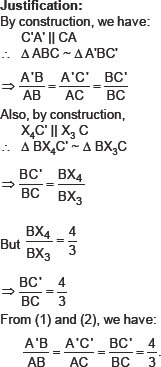Q.7.   Draw a right triangle in which the sides (other than hypotenuse) are of lengths 4 an and 3 cm. Then construct another triangle whose sides are 5 times the corresponding sides of the given triangle.
Sol. Steps of construction: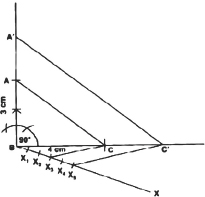I. Construct the right triangle ABC such that ∠B = 90°, BC = 4 cm and BA = 3 cm.
II. Draw a ray BX such that an acute angle ∠CBX is formed.
III. Mark 5 points X1, X2, X3, X4 and X5 on BX such that
BX1 = X1X2 = X2X3 = X3X4 = X4X5.
IV. Join X3 to C.
V. Draw a line through X5 parallel to X3 C, intersecting the extended line segment BC at C'.
VI. Draw another line through C' parallel to CA intersecting the extended line segment BA at A'.
Thus, Δ A' BC' is the required triangle.
Justification:
By construction, we have: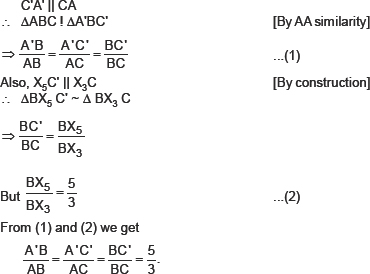EXERCISE 11.2
Q.1.   In each of the following, give also the justification of the construction:
Draw a circle of radius 6 cm. From a point 10 cm away from its centre, construct the pair of tangents to the circle and measure their lengths.
Sol. Steps of construction:
I. With O as centre and radius 6 cm, draw a circle.
II. Take a point P at 10 cm away from the centre.
III. Join O and P.
IV. Bisect OP at M.
V. Taking M as centre and MP or MO as radius, draw a circle.
VI. Let the new circle intersects the given circle at A and B.
VII.Join PA and PB.
Thus, PA and PB are the required two tangents.
By measurement, we have:
PA = PB = 9.6 cm.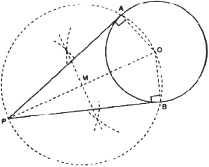Justification:
Join OA and OB
Since PO is a diameter.
∴ ∠OAF = 90° = ∠OBP                                [Angles in a semicircle]
Also, OA and OR are radii of the same circle.
⇒ PA and PB are tangents to the circle.
Q.2.   Construct a tangent to a circle of radius 4 cm from a point on the concentric and measure its length. Also verify the measurement by actual calculation.
Sol. Steps of construction: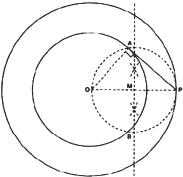I. Join PO and bisect it such that the mid point of PO is represented by M.
II. Taking M as centre and OM or MP as radius, draw a circle such that this circle intersects the circle (of radius 4 cm) at A and B.
III. Join A and P.
Thus, PA is the required tangent. By measurement, we have:
PA = 4.5 cm
Justification:
Join OA such that
∠PAO = 90° [Angle in a semi-circle]
⇒ PA ⊥ OA
∵ OA is a radius of the inner circle.
∴ PA has to be a tangent to the inner circle.
Q.3.   Draw a circle of radius 3 cm. Take two points P and Q on one of its extended diameters each at a distance of 7 an from its centre. Draw tangents to the circle from these two points P and Q.
Sol. Steps of construction:
I. Join P and O.
II. Bisect PO such that M be its mid-point.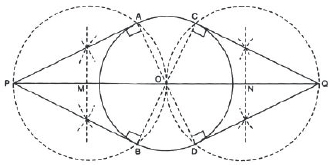III. Taking M as centre and MO as radius, draw a circle. Let it at A and B.
IV. Join PA and PB.
Thus, PA and PB are the two required tangents from P.
V. Now, join O and Q.
VI. Bisect OQ such that N is its mid point.
VII.Taking N as centre and NO as radius, draw a circle. Let it at C and D.
VIII. Join QC and QD.
Thus, QC and QD are the required tangents to the given
Justification:
Join OA such that ∠OAP = 90°                                          [Angle in a semi-circle]
⇒ PA ⊥ OA PA is a tangent.
Similarly, PB ⊥ OA ⇒ PB is a tangent
Now, join OC such that ∠QCO = 90°                                                        [Angle in a semi-circle]
⇒ QC ⊥ OC ⇒ QC is a tangent.
Similarly, QD ⊥ OC ⇒ QD is a tangent.
Q.4.   Draw a pair of tangents to a circle of radius 5 cm which are inclined to each other at an angle of 60°.
Sol. Steps of construction:
I. With centre O and radius = 5 cm, draw a circle.
II. Draw an angle ∠AOB = 120°.
III. Draw a perpendicular on OA at A.
IV. Draw another perpendicular on OB at B.
V. Let the two perpendiculars. meet at C.
CA and CB are the two required tangents to the given circle which are inclined to each other at 60°.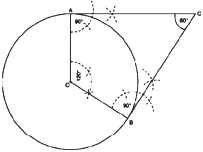Justification:
In a quadrilateral OACB, using angle sum property, we have:
120° + 90° + 90° + ∠ACE = 360°
⇒ 300 + ∠ACE = 360°
⇒ ∠ACB = 360° – 300° = 60°.
Q.5.   Draw a line segment AB of length 8 an. Taking A as centre, draw a circle of radius 4 cm and taking B as centre, draw another circle of radius 3 cm. Construct tangents to each circle from the centre of the other circle.
Sol. Steps of construction: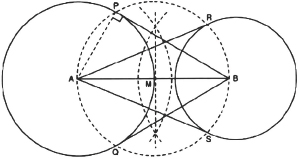I. Bisect the line segment AB. Let its mid point be M.
II. With centre as M and MA (or MB) as radius, draw a circle such that it intersects the circle with centre A at the points P and Q.
III. Join BP and BQ.
Thus, BP and BQ are the required two tangents from B to the circle with centre A.
IV. Let the circle with centre M, intersects the circle with centre B at R and S.
V. Join RA and SA.
Thus, RA and SA are the required two tangents from A to the circle with centre B.
Justification:
Let us join A and P.
∵ ∠APB = 90°                                          [Angle in a semi circle]
∴ BP ⊥ AP
But AP is radius of the circle with centre A.
⇒ BP has to be a tangent to the circle with centre A.
Similarly, BQ has to be tangent to the circle with centre A.
Also, AR and AS have to be tangent to the circle with centre B.
Q.6.   Let ABC be a right triangle in which AB = 6 cm, BC = 8 an and LB = 90°. BD is the perpendicular from B on AC. The circle through B, C, D is drawn. Construct the tangents from A to this circle. [CBSE 2012)
Sol. Steps of construction:
I. Join AO (0 is the centre of the circle passing through B, C and D.)
II. Bisect AO. Let M be the mid point of AO.
III. Taking M as centre and MA as radius, draw a circle intersecting the given circle at B and E.
IV. Join AB and AE. Thus, AB and AE are the required two tangents to the given circle from A.
Justification:
Join OE, then ∠AEO = 90°                                          [Angle being in a semi circle]
∴ AE ⊥ OE.
But OE is a radius of the given circle.
⇒ AE has to be a tangent to the circle.
Similarly, AB is also a tangent to the given circle.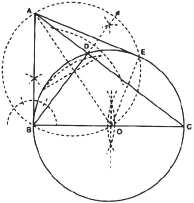Q.7.   Draw a circle with the help of a bangle. Take tangents from this point to the circle.
Sol. Steps of construction:
I. Draw the given circle using a bangle.
II. Take two non parallel chords PQ and RS of this circle.
III. Draw the perpendicular bisectors of PQ and RS such that they intersect at O. Therefore, O is the centre of the given circle.
IV. Take a point P' outside this circle.
V. Join OP' and bisect it. Let M be the mid point of OP'.
VI. Taking M as centre and OM as radius, draw a circle. Let it intersect the given circle at A and B.
VII.Join P'A and P'B. Thus, P'A and P'B are the required two tangents.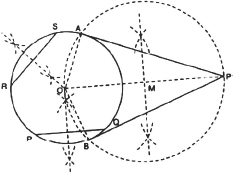Justification:
Join OA and OB.
Since ∠OAP = 90°
∴ PA ⊥ OA